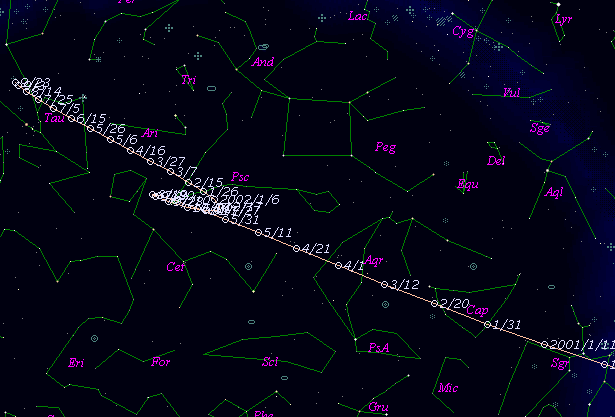# 71P/Clark (2000)###Orbital Elements

```   The following improved orbital elements by Kenji Muraoka,
are from 191 observations 1989 to 2000, including 9 Planets,
Moon and 5 minor planets perturbations and non-gravitational
effect of style II.  The mean residual is +/- 0.90 arc seconds.

Epoch  =  2000 Dec.  2.0  TT       JDT = 2451880.5
T  =  2000 Dec.  2.04069       +/- 0.00308 (m.e.) TT
Peri. =  208.84748                +/- 0.00070
Node  =   59.69248                +/- 0.00010   (2000.0)
Incl. =    9.49522                +/- 0.00002
q  =    1.5591537              +/- 0.0000024 AU
e  =    0.5005353              +/- 0.0000003
a  =    3.1216492              +/- 0.0000017 AU
n  =    0.17870139             +/- 0.00000015
P  =    5.515                  +/- 0.0000045  years
A1  =   +0.167                  +/- 0.077
A2  =   -0.45441                +/- 0.00202
```

###Finding Charts###Magnitudes Graph

```        m1 = 6.0 + 5 log d + 25.0 log r(t - 30)
```##### The orbital elements are calculated by Kenji Muraoka. The charts are made with StellaNavigator Ver.2.0 for Windows (AstroArts / ASCII). The magnitudes graphs are made with Comet for Windows.